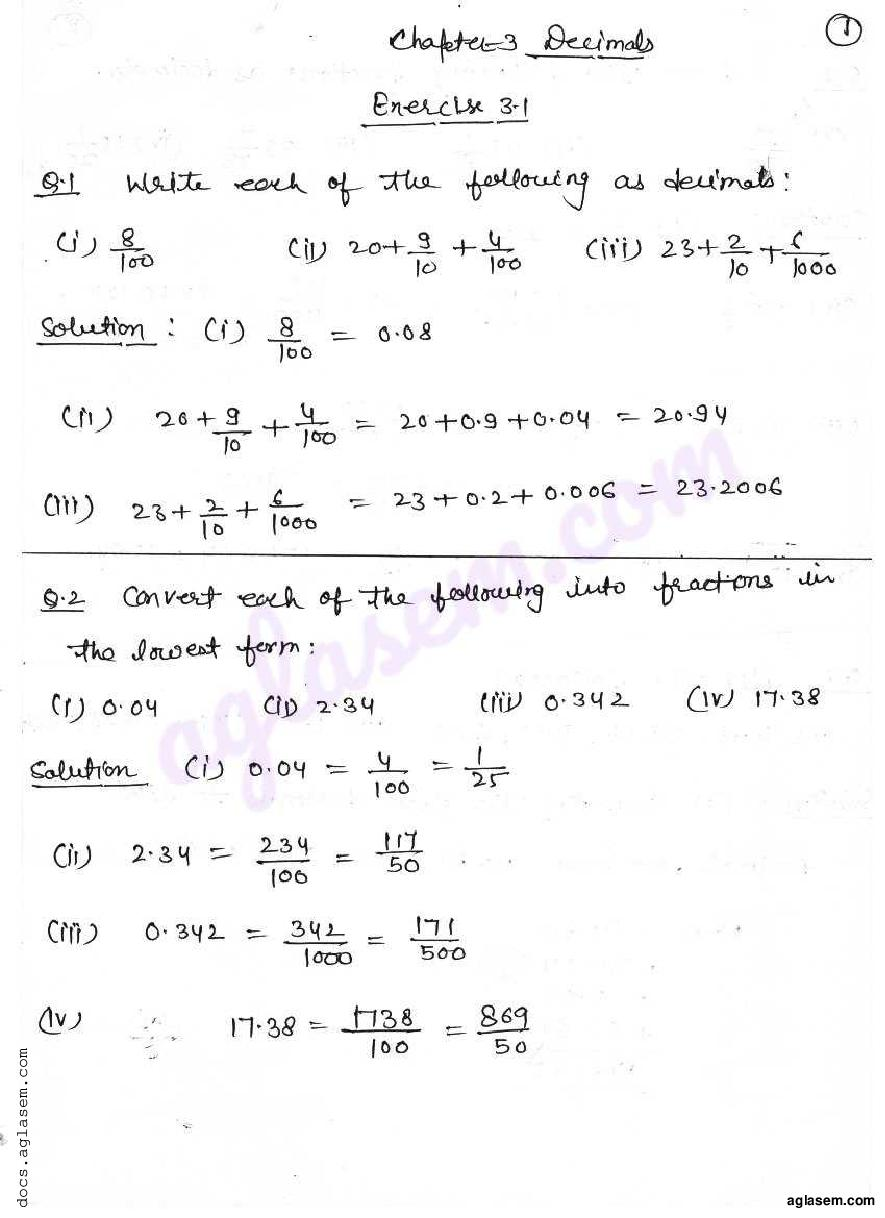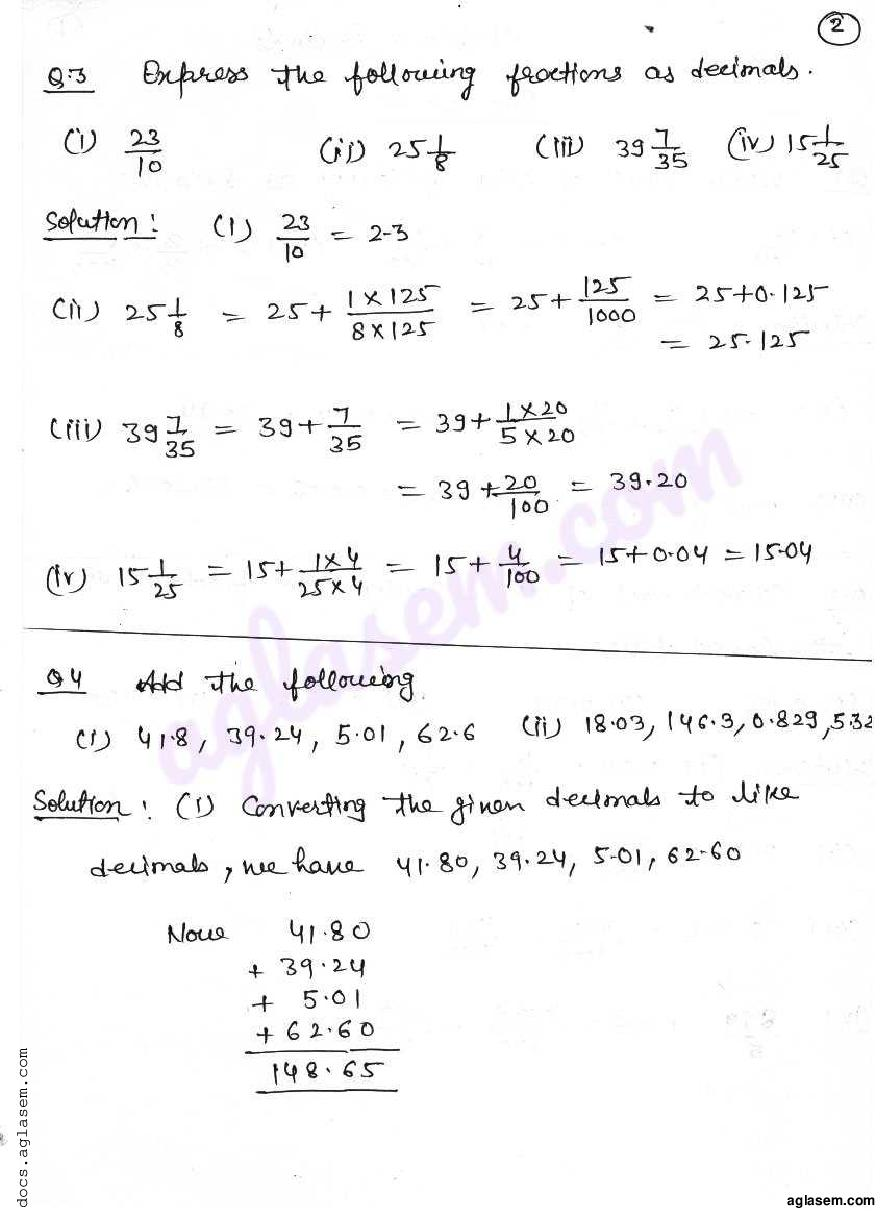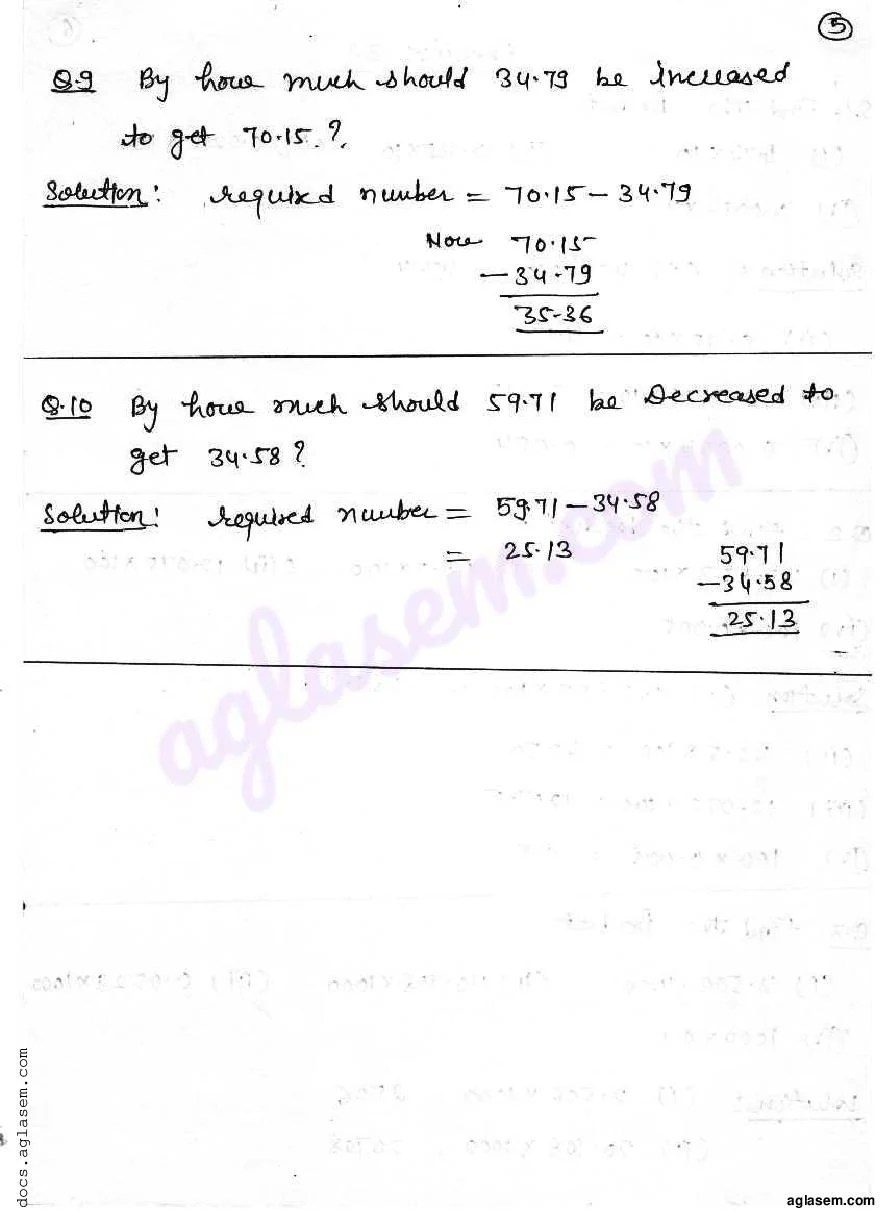# RD Sharma Solutions Class 7 Chapter 3 Decimals Exercise 3.1

Here you can get free RD Sharma Solutions for Class 7 Maths Chapter 3 Decimals Exercise 3.1. All RD Sharma Book Solutions are given here exercise wise for the chapter Decimals. RD Sharma Solutions are helpful in the preparation of several school level, graduate and undergraduate level competitive exams. Practicing questions from RD Sharma Mathematics Solutions for Class 7 Chapter 3 Decimals is proven to enhance your math skills.

 Class: Class 7th Chapter: Chapter 3 Exercise: Exercise 3.1 Name: Decimals

## RD Sharma Solutions Class 7 Chapter 3 Decimals Exercise 3.1

RD Sharma Class 7 Solutions Chapter 3 for Decimals Exercise 3.1 are given below.

RD Sharma Solutions Class 7 Chapter 3 Decimals Exercise 3.1